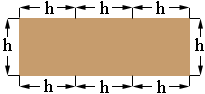SEARCH HOMEMath Central Quandaries & QueriesSubject: geometry Name: mike Who are you: Parent The perimeter of a rectangle is 72 in. The base is 3 times the height. find the area of the rectangle?Hi Mike,

I let h be the height of the rectangle and drew a sketch.From the diagram you can see that the perimeter is 8h and hence

8h = 72

PennyMath Central is supported by the University of Regina and The Pacific Institute for the Mathematical Sciences.